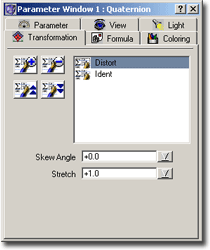### Quaternion – Transformation ParametersThe transformation tab lets you add transformations to the Quaternion. For more information about transformation formulas and their meaning see Transformations.

You add transformations by double clicking onto a transformation formula in the compiler window or by selecting a transformation formula in the compiler window and pressing the “Add Transformation” image button in the parameter window.

Of course, you should assign only Quaternion Transformations to a Quaternion, because only such transformations are prepared to obtain a quaternion number and return a quaternion number. Most other transformations won’t work because they use illegal operations.

Because a transformation simply “transforms” (or, in other words, takes a quaternion number and produces a quaternion number), it is possible to apply several transformations: The succeeding transformation takes as input the output from the preceding transformation.

And this indeed makes sense: Suppose you have a simple transformation which just “moves” the input area (takes the input value and adds a quaternion number). Suppose you also have a transformation which does some rotation. If you now want to combine these two transformations you could write a more complicated transformation which “moves” and “rotates”. But wouldn’t it be easier, if you just apply the “movement” transformation, and after that apply the “rotation” transformation? ChaosPro allows you to do that: You can write many simple understandable transformations. And the user who simply wants to create fractals can combine any number of transformations to obtain interesting sets of the complex number plane. For more information about setting parameters of your transformations see Setting Formula Parameters.